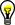# Batch Results

After you have trained or classified a folder of images, the Batch Results dialog box displays the results of the batch training. The results are displayed on five tabs: Classification Results, Scores Histogram, Class Information, Classification Distribution, and Distance Table.

## Classification Results Tab

The classification results are displayed in a chart that lists the following results for each file trained and classified:

• Label—Class to which the sample belongs.
• Classification Score—Degree of certainty that a sample is assigned to one class instead of other classes.
• Identification Score—Degree of similarity between a sample and members of the class to which the sample is assigned.
• Classification—Classification is Good if the sample label found during the classification process matches the label provided by the user. The classification is Bad if the sample label does not match.

## Scores Histogram Tab

The scores histogram displays the number of each sample classified and trained for each score value.

The X-axis can represent either classification scores or identification scores. The Histogram Mode can be either linear or cumulative.

## Class Information Tab

The accuracy and predictive value of the classification are displayed in a chart for each class.

• Samples—Number of samples belonging to the class.
• Standard Deviation—Standard deviation from the mean of all samples in the class.
• Accuracy (sensitivity)—Probability that a sample is classified into the class to which it belongs.
• Predictive Value—Probability that a sample classified into a given class belongs to that class.

## Classification Distribution Tab

The classification distribution is displayed in a chart, and the classifier accuracy is calculated. The classification distribution is a matrix that gives the probability that a sample belonging to a specific class will be classified in another class.

## Distance Table Tab

The distance table displays the mean distance from each class to each other class.Tip  You can also display this dialog by choosing Tools»Training Summary. Only the Class Information, Classification Distribution, and Distance Table tabs are available.